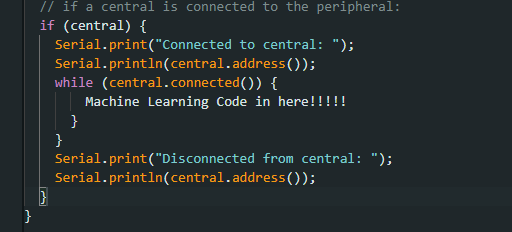# Interface with BLE

After deploy the code to arduino, I found that there is somethings interfering with the BLE.

I combined the arduinoBLE library and the machine learning library for transfer data. However, the connecting time of BLE is so long, it takes around 10s to show the word “connected” in nRF connect app.The machine learning code is placed in here. When I click the connect button, the model will run immediately and keep showing the result in the Serial monitor. But the BLE will keep showing “connecting” in the same time until 10s after to finish the connection.

After checking the code, the problem is because the “delayMicroseconds(next_tick - micros());” in the for loop.

``````for (size_t ix = 0; ix < EI_CLASSIFIER_DSP_INPUT_FRAME_SIZE; ix += 3) {
// Determine the next tick (and then sleep later)
uint64_t next_tick = micros() + (EI_CLASSIFIER_INTERVAL_MS * 1000);

IMU.readAcceleration(buffer[ix], buffer[ix + 1], buffer[ix + 2]);

for (int i = 0; i < 3; i++) {
if (fabs(buffer[ix + i]) > MAX_ACCEPTED_RANGE) {
buffer[ix + i] = ei_get_sign(buffer[ix + i]) * MAX_ACCEPTED_RANGE;
}
}

buffer[ix + 0] *= CONVERT_G_TO_MS2;
buffer[ix + 1] *= CONVERT_G_TO_MS2;
buffer[ix + 2] *= CONVERT_G_TO_MS2;

delayMicroseconds(next_tick - micros());
}

``````

If I just comment it, the learning model will start next sample so fast, it may affect the result. How can i solve it? The original time is 2second per 1 classification. After delete it, it become 0.3second per 1